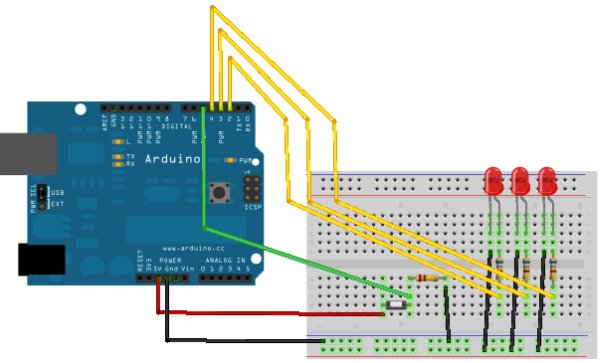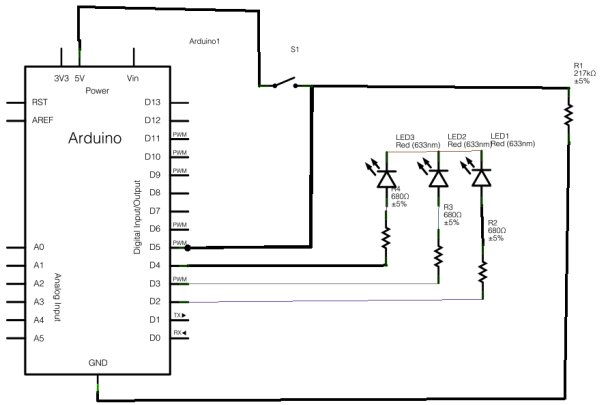# Arduino Binary Die using arduino

After buying a Nanode (an Arduino-compatible board with ethernet built-in) last weekend, we’ve been trying to work it out by making a couple of simple examples, the ‘Binary Dice’ is the first one with input and outputs.

A note, this code example is based on the one from the book Arduino: A Quick-Start Guide by Maik Schmidt.

You’ll need (other than the Nanode/Arduino board):• 3 LEDs (ours were rated for 0.8mA-0.12mA at 6V)
• 3 Resistors to bring the 5V down to a reason current for the LEDs (we used 680Ω)
• 1 large resistor to flatten out the fluctuations in the ground rail (we used 17KΩ)
• A push-to-make button

## Arduino Sketch

You may need to change the constants at the beginning to match your board and where you plug things in.

```const unsigned int LED_BIT0 = 4;
const unsigned int LED_BIT1 = 2;
const unsigned int LED_BIT2 = 3;
const unsigned int BUTTON_PIN = 5;
const unsigned int BAUD_RATE = 19200;

void setup() {
pinMode(LED_BIT0, OUTPUT);
pinMode(LED_BIT1, OUTPUT);
pinMode(LED_BIT2, OUTPUT);
pinMode(BUTTON_PIN, INPUT);
Serial.begin(BAUD_RATE);
}

void loop() {

if (CURRENT_BUTTON_STATE == HIGH || command == '1') {
reset_LEDs();
delay(1000);
long result = random(1, 7);
output_result(result);
}
}void reset_LEDs() {
digitalWrite(LED_BIT0, LOW);
digitalWrite(LED_BIT1, LOW);
digitalWrite(LED_BIT2, LOW);
}

void output_result(const long result) {
digitalWrite(LED_BIT0, result & B001);
digitalWrite(LED_BIT1, result & B010);
digitalWrite(LED_BIT2, result & B100);
Serial.print(result)
}```

For more detail: Arduino Binary Die